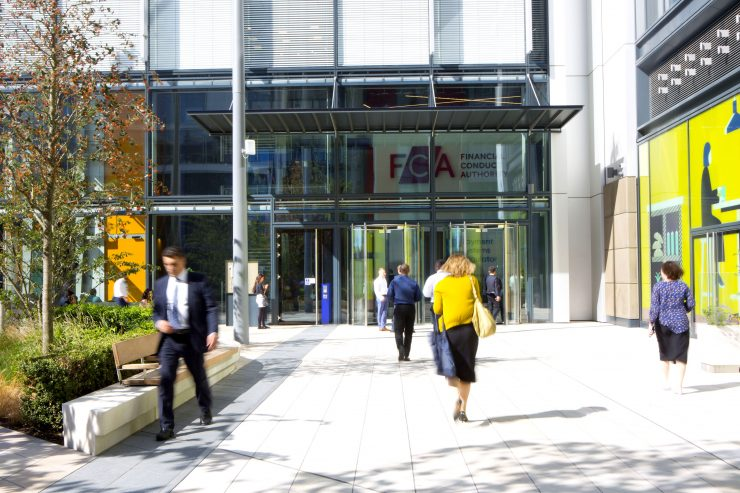# FCA publishes information on APRC calculations

FCA has published information on its website relating to the calculation of the Annual Percentage Rate of Charge (APRC) which lenders use for disclosing associated costs of a mortgage contract to borrowers. The figure will show, as a percentage, the annual cost of a mortgage over its lifetime. However, systems issues have meant that some firms have incorrectly calculated the amount following the market volatility in 2022. Problems occurred when firms were calculating an APRC for a mortgage where the reversion rate is lower than the initial introductory rate period.

FCA says that where this is the case, firms should comply with MCOB 10A.3.1R(5) and calculate the APRC on the basis that the initial rate will continue until the end of the term. It says 88% of mortgages in late 2022 were priced with an introductory rate higher than the reversion rate, whereas less than 5% of products are currently priced in that way. It expects firms to take prompt action to ensure any wrong calculations are corrected as soon as possible.  Although, in general, an APRC calculated on incorrect assumptions is unlikely of itself to deprive a borrower of the ability to make an informed choice, there does need to be consistency across calculations to help to make comparisons.